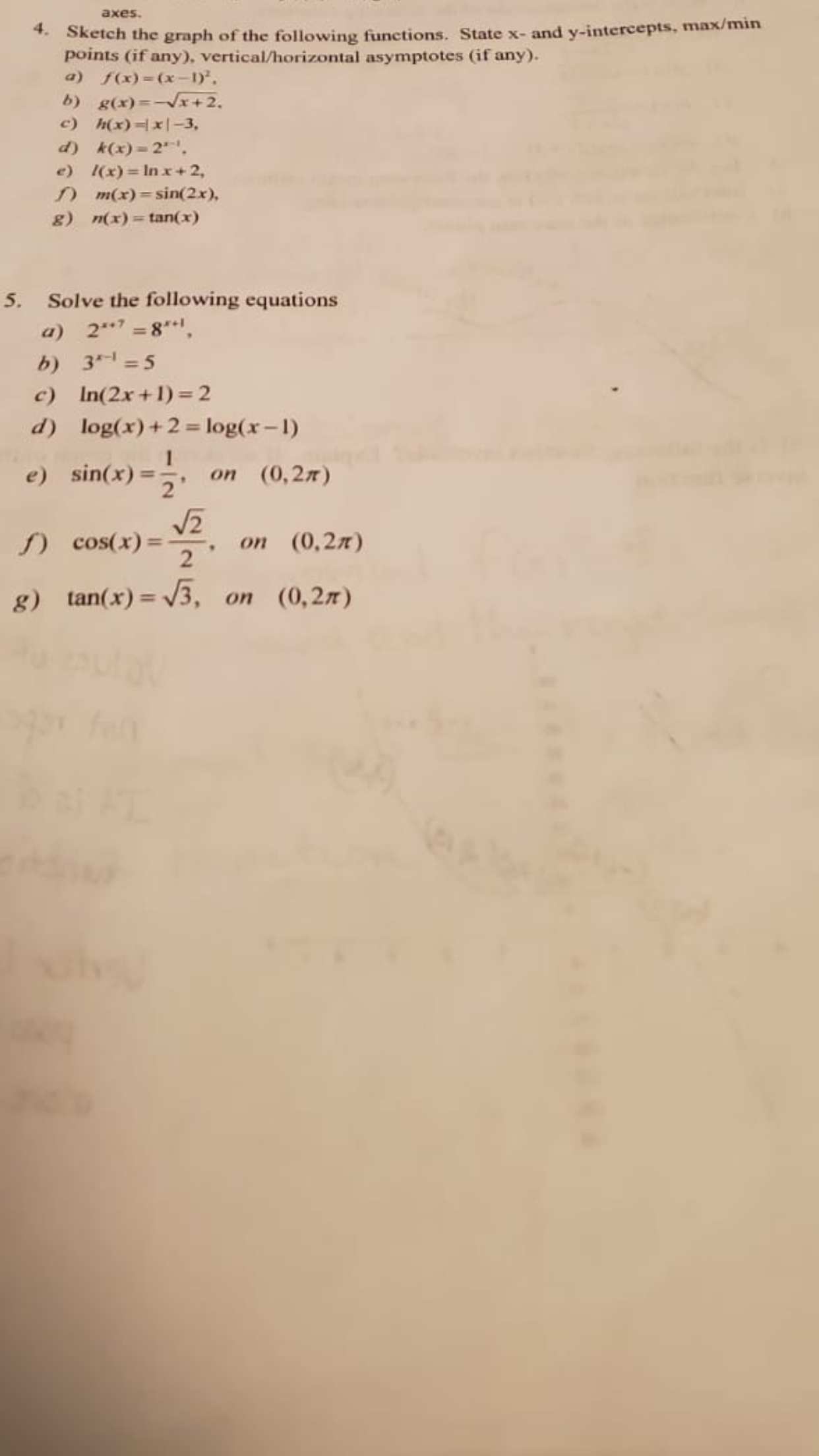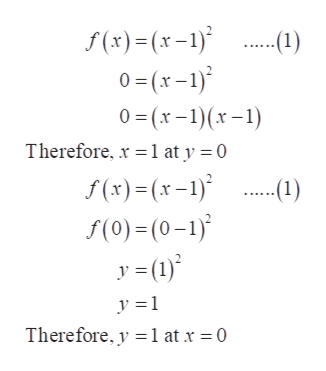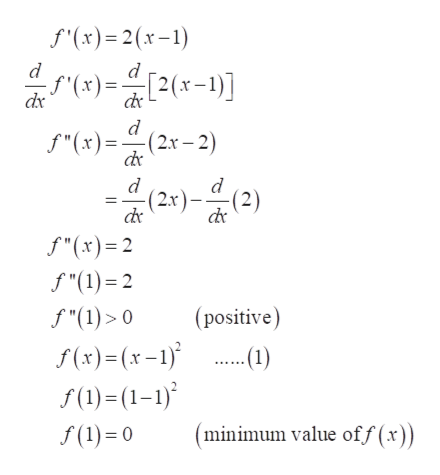# axes.** Sketch the graph of the following functions. State x- and y-intercepts, max/minpoints (if any). vertical/horizontal asymptotes (if any).a) f(x) = (x-1).b) g(x)=-Vx+2,c) h(x)=|x|-3,d) k(x) = 2.e) (x)= In x+ 2,n m(x) = sin(2x),g)n(x) = tan(x)5. Solve the following equationsa) 2**7 = 8,b) 3 =5c) In(2x+1)= 2d) log(x)+2= log(x-1)e) sin(x) =, on (0,27)%3D) cos(x) =оn (0,2л)%3Dg) tan(x) = V3, on (0,27)%3D

Question
19 viewshelp_outlineImage Transcriptioncloseaxes. ** Sketch the graph of the following functions. State x- and y-intercepts, max/min points (if any). vertical/horizontal asymptotes (if any). a) f(x) = (x-1). b) g(x)=-Vx+2, c) h(x)=|x|-3, d) k(x) = 2. e) (x)= In x+ 2, n m(x) = sin(2x), g) n(x) = tan(x) 5. Solve the following equations a) 2**7 = 8, b) 3 =5 c) In(2x+1)= 2 d) log(x)+2= log(x-1) e) sin(x) =, on (0,27) %3D ) cos(x) = оn (0,2л) %3D g) tan(x) = V3, on (0,27) %3D fullscreen
check_circle

Step 1

Hello. Since your question has multiple sub-parts, we will solve the first three sub-parts for you. If you want remaining sub-parts to be solved, then please resubmit the whole question and specify those sub-parts you want us to solve.

Hence, solving a, b and c.

(a)

To find the intercept of x put f (x) = 0 in f (x) and solve for x.

Similarly, to find the y-intercept put x = 0 in equation (1) and solve for f (0).help_outlineImage Transcriptionclosef (x)=(x-1)° 0= (x-1) 0 =(x-1)(x–1) -.(1) Therefore, x = 1 at y = 0 S(x)=(x -1)* f(0)=(0-1) y = (1)* ...(1) y =1 Therefore, y =1 at x = 0 fullscreen
Step 2

Now, to find the minimum and maximum for f (x), find the critical points for f (x).

First differentiate f (x) w.r.t x and get f '(x) and then put f '(x) = 0 and find the value for x.

The value of x will be the critical point.help_outlineImage Transcriptionclosef (x)=(x-1) .(1) f (x)=(x-1) de de f"(x)= 2(x-1)-(x-1) dx = 2(x-1)(x-1) dx -기남마을이 2(x-1) (x)-(1) = dx de = 2(x-1)[1-0] f'(x) = 2(x-1) Now, f '(x)= 0 2(x-1)=0 (x-1)=0 (critical point) r= 1 fullscreen
Step 3

Then, again differentiate f '(x) w.r.t x and get f ''(x) and then find the value of f ''(1) because x = 1 is the critical point.

As the value of f ''(1) is positive therefore, f (x) will be minimum at x = 1.

Put the value of x in f (x) as 1 and find f (1). f (1) will be the minimum value of f (x).

Now, to find the asymptote observe ...help_outlineImage Transcriptionclosef'(x)= 2(x-1) d (x) = [2(x-1)] f"(x)= f"(x)=(2x– 2) (2+)-(2) (2.x)- de de f"(x)=2 f "(1) = 2 (positive) f "(1) > 0 f (x)=(x-1) f (1)=(1–1) f (1) = 0 -(1) (minimum value of f (x)) I| || fullscreen

### Want to see the full answer?

See Solution

#### Want to see this answer and more?

Solutions are written by subject experts who are available 24/7. Questions are typically answered within 1 hour.*

See Solution
*Response times may vary by subject and question.
Tagged in

### Functions